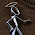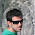## Friday, August 10, 2012

### MurMurHash3, an ultra fast hash algorithm for C# / .NET

Finding good hash functions for larger data sets is always challenging. The well know hashes, such as MD5, SHA1, SHA256 are fairly slow with large data processing and their added extra functions (such as being cryptographic hashes) isn’t always required either.

Performance and low collision rate on the other hand is very important, so many new hash functions were inverted in the past few years. One of the most notable ones is MurMurHash3, which is an improved version of its predecessor (v2). The new version can create both 32 bit and 128 bit hash values, making it suitable for a wide range of applications.

64 bit architecture

The 32 bit hash isn’t too important for large amount of data matching, so I only worked with and tested the 128 bit version. The latter one is optimized for x86-64 architecture, giving amazing speeds for data processing. As a 128 bit value consists of two 64 integers, the processor is fairly good with working with such large hash values – they are not byte arrays but represented as two longs!

PerformanceHere is a short comparison between the well know hash functions, running on a 64 bit platform. It’s fairly obvious that MurMur Hash 3 easily outperforms the built-in .NET 4.0 hash functions.

When to use it

In every scenario when we need to find two or more matching byte arrays – documents, images, text files, email messages, etc. In this case, the 128 bit fingerprint and low collision rate provides excellent matching for the samples. A nice use-case is identifying then removing spam messages every mailboxes in the domain. As the number of messages is huge, searching by content is not always feasible. On the other hand, creating and matching their hash values is lightning fast, so all we need to do is find a message bit it’s hash value – which can be a database key for instance.

When NOT to use it

As MurMur3 creates a fairly big print (128 bits), so it’s not recommended to use it for hashing small data sets like words, short strings, integers and so on. The embedded hash functions for these simple and small data types provide excellent performance with low collision rate. So anything that is less than a couple dozen, or preferably hundreds of bytes is not a good candidate.

MurMurHash3 source code in C#

The code uses unsafe operation, so turn on this flag when compiling. The best performance can be achieved on the 64 bit platforms so make sure to enable 64 bit builds too.
To calculate the hash of a byte array, simply call ComputeHash.

```class Murmur3
{
// 128 bit output, 64 bit platform version

public static ulong READ_SIZE = 16;
private static ulong C1 = 0x87c37b91114253d5L;
private static ulong C2 = 0x4cf5ad432745937fL;

private ulong length;
private uint seed; // if want to start with a seed, create a constructor
ulong h1;
ulong h2;

private void MixBody(ulong k1, ulong k2)
{
h1 ^= MixKey1(k1);

h1 = h1.RotateLeft(27);
h1 += h2;
h1 = h1 * 5 + 0x52dce729;

h2 ^= MixKey2(k2);

h2 = h2.RotateLeft(31);
h2 += h1;
h2 = h2 * 5 + 0x38495ab5;
}

private static ulong MixKey1(ulong k1)
{
k1 *= C1;
k1 = k1.RotateLeft(31);
k1 *= C2;
return k1;
}

private static ulong MixKey2(ulong k2)
{
k2 *= C2;
k2 = k2.RotateLeft(33);
k2 *= C1;
return k2;
}

private static ulong MixFinal(ulong k)
{
// avalanche bits

k ^= k >> 33;
k *= 0xff51afd7ed558ccdL;
k ^= k >> 33;
k *= 0xc4ceb9fe1a85ec53L;
k ^= k >> 33;
return k;
}

public byte[] ComputeHash(byte[] bb)
{
ProcessBytes(bb);
return Hash;
}

private void ProcessBytes(byte[] bb)
{
h1 = seed;
this.length = 0L;

int pos = 0;
ulong remaining = (ulong)bb.Length;

// read 128 bits, 16 bytes, 2 longs in eacy cycle
{
ulong k1 = bb.GetUInt64(pos);
pos += 8;

ulong k2 = bb.GetUInt64(pos);
pos += 8;

MixBody(k1, k2);
}

// if the input MOD 16 != 0
if (remaining > 0)
ProcessBytesRemaining(bb, remaining, pos);
}

private void ProcessBytesRemaining(byte[] bb, ulong remaining, int pos)
{
ulong k1 = 0;
ulong k2 = 0;
length += remaining;

// little endian (x86) processing
switch (remaining)
{
case 15:
k2 ^= (ulong)bb[pos + 14] << 48; // fall through
goto case 14;
case 14:
k2 ^= (ulong)bb[pos + 13] << 40; // fall through
goto case 13;
case 13:
k2 ^= (ulong)bb[pos + 12] << 32; // fall through
goto case 12;
case 12:
k2 ^= (ulong)bb[pos + 11] << 24; // fall through
goto case 11;
case 11:
k2 ^= (ulong)bb[pos + 10] << 16; // fall through
goto case 10;
case 10:
k2 ^= (ulong)bb[pos + 9] << 8; // fall through
goto case 9;
case 9:
k2 ^= (ulong)bb[pos + 8]; // fall through
goto case 8;
case 8:
k1 ^= bb.GetUInt64(pos);
break;
case 7:
k1 ^= (ulong)bb[pos + 6] << 48; // fall through
goto case 6;
case 6:
k1 ^= (ulong)bb[pos + 5] << 40; // fall through
goto case 5;
case 5:
k1 ^= (ulong)bb[pos + 4] << 32; // fall through
goto case 4;
case 4:
k1 ^= (ulong)bb[pos + 3] << 24; // fall through
goto case 3;
case 3:
k1 ^= (ulong)bb[pos + 2] << 16; // fall through
goto case 2;
case 2:
k1 ^= (ulong)bb[pos + 1] << 8; // fall through
goto case 1;
case 1:
k1 ^= (ulong)bb[pos]; // fall through
break;
default:
throw new Exception("Something went wrong with remaining bytes calculation.");
}

h1 ^= MixKey1(k1);
h2 ^= MixKey2(k2);
}

public byte[] Hash
{
get
{
h1 ^= length;
h2 ^= length;

h1 += h2;
h2 += h1;

h1 = Murmur3.MixFinal(h1);
h2 = Murmur3.MixFinal(h2);

h1 += h2;
h2 += h1;

Array.Copy(BitConverter.GetBytes(h1), 0, hash, 0, 8);
Array.Copy(BitConverter.GetBytes(h2), 0, hash, 8, 8);

return hash;
}
}
}
```

Some helper functions for reading 64 bit integers from a byte array and rotating unsigned longs:

```public static class IntHelpers
{
public static ulong RotateLeft(this ulong original, int bits)
{
return (original << bits) | (original >> (64 - bits));
}

public static ulong RotateRight(this ulong original, int bits)
{
return (original >> bits) | (original << (64 - bits));
}

unsafe public static ulong GetUInt64(this byte[] bb, int pos)
{
// we only read aligned longs, so a simple casting is enough
fixed (byte* pbyte = &bb[pos])
{
return *((ulong*)pbyte);
}
}
}
```

1.You don't need unsafe code. Use this.

public static ulong GetUInt64(this byte[] bb, int pos)
{
return BitConverter.ToUInt64(bb, pos);
}

1.That is correct, you don't need it but if you want performance, you'll want it.

The simple unsafe functions above will be inlined (faster) while an external library call will always be expensive (slower).

Moreover, the BitConverter implementation is full of checks for edge cases which I don't have, making that implementation much slower (but much safer too). By the way, the BitConverter uses unsafe too.

2.For whatever reason it's coming in twice as slow as djb2hash. Any guesses?

2.One small mod is to set h2=0 at the top of ProcessBytes(). By doing so, you allow the object to be re-used instead of being a one-use object.

3.Any chance of modifying this to work on data bigger than uint ??
I would like to use it as a MD5 replacement for large data files, just note sure whats the optimal way to modify the code above.
Thanks.

4.question: is this code thread safe?
does creating 2 different instances in 2 different threads assures that the 2 results won't be affected by each other?

1.After a quick look - the code is not inherently threadsafe. While two separate instances would not themselves affect each other, it is possible for the object being hashed to change during operation, and therefore it is not threadsafe.

In addition, the "READ_SIZE" variable is not readonly, so it can break ALL instances if it is altered.

Making READ_SIZE readonly, or non-static, plus a small change to the "computeHash" should be enough to make it threadsafe, I believe.

2.5.I love your implementation congratulations! But, there is a way to pass a Stream in input?

6.To remove the unsafe requirement but not use BitConverter you can do:

return (UInt32)(bb[pos++] | bb[pos++] << 8 | bb[pos++] << 16 | bb[pos++] << 24);

7.VS2017 error:
Error CS1061 "ulong" does not contain a definition for "RotateLeft", and could not find an available extension method "RotateLeft" that takes the type "ulong" as the first argument (possibly using a directive using or a link to the assembly).